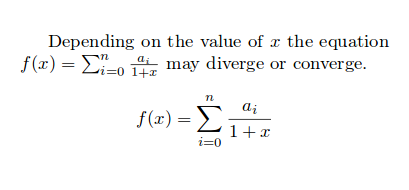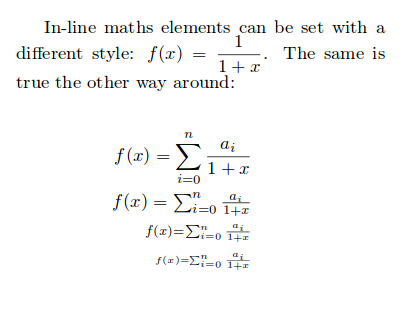Showing first {{hits.length}} results of {{hits_total}} for {{searchQueryText}}{{hits.length}} results for {{searchQueryText}}

No Search Results

Some mathematical elements change their style depending on the context, whether they are in line with the text or in an equation-type environment. This article explains how to manually adjust the display style. Introduction Let's see an example Depending on the value of $x$ the equation $$f(x) = \sum_{i=0}^{n} \frac{a_i}{1+x}$$ may diverge or converge. $f(x) = \sum_{i=0}^{n} \frac{a_i}{1+x}$Superscripts, subscripts and fractions are formatted differently.   Open an example in Overleaf Setting mathematical styles The maths styles can be set explicitly. For instance, if you want an in-line mathematical element to display as a equation-like element put \displaystyle before that element. There are some more maths style-related commands that change the size of the text. In-line maths elements can be set with a different style: $$f(x) = \displaystyle \frac{1}{1+x}$$. The same is true the other way around: \begin{eqnarray*} \begin{eqnarray*} f(x) = \sum_{i=0}^{n} \frac{a_i}{1+x} \\ \textstyle f(x) = \textstyle \sum_{i=0}^{n} \frac{a_i}{1+x} \\ \scriptstyle f(x) = \scriptstyle \sum_{i=0}^{n} \frac{a_i}{1+x} \\ \scriptscriptstyle f(x) = \scriptscriptstyle \sum_{i=0}^{n} \frac{a_i}{1+x} \end{eqnarray*} \end{eqnarray*}Open an example in Overleaf Further reading For more information see Mathematical expressions Subscripts and superscripts Aligning equations with amsmath Spacing in math mode Integrals, sums and limits Mathematical fonts The not so short introduction to LaTeX2ε